# GMAT Math : DSQ: Understanding intersecting lines

## Example Questions

### Example Question #141 : Data Sufficiency Questions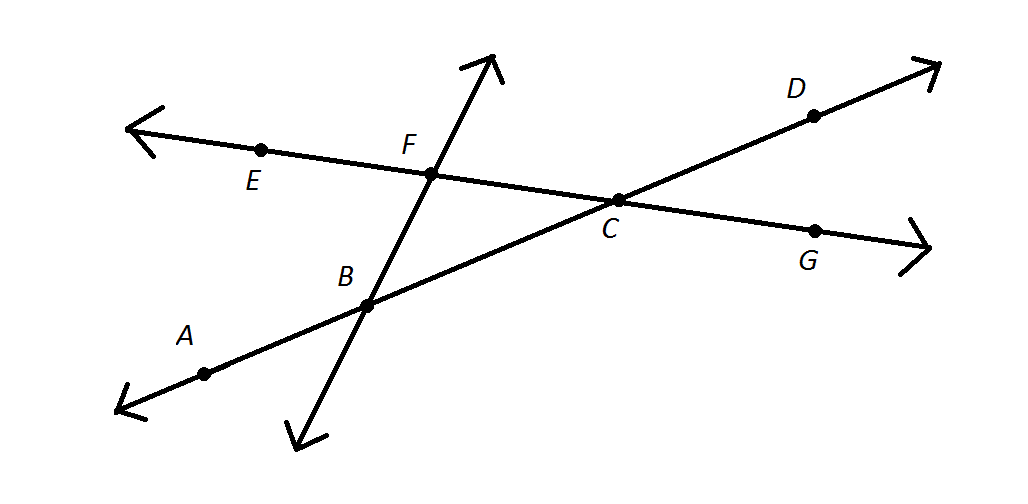Refer to the above figure. Jane chose one of the line segments shown in the above diagram but she will not reveal which one. Which one did she choose?

Statement 1: One of the endpoints of the line segment is.

Statement 2: The line segment includes.

BOTH statements TOGETHER are sufficient to answer the question, but NEITHER statement ALONE is sufficient to answer the question.

EITHER statement ALONE is sufficient to answer the question.

Statement 2 ALONE is sufficient to answer the question, but Statement 1 ALONE is NOT sufficient to answer the question.

Statement 1 ALONE is sufficient to answer the question, but Statement 2 ALONE is NOT sufficient to answer the question.

BOTH statements TOGETHER are insufficient to answer the question.

BOTH statements TOGETHER are insufficient to answer the question.

Explanation:

If we know both statements, then we know that the segment can be either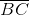or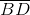, since each has endpointand each includes; we can not eliminate either, however.

### Example Question #2 : Dsq: Understanding Intersecting Lines

How many times doesand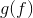intersect?

I)is a linear equation with a slope of.

II)is quadratic equation with a vertex at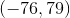.

Statement I is sufficient to answer the question, but statement II is not sufficient to answer the question.

Both statements are needed to answer the question.

Either statement is sufficient to answer the question.

Statement II is sufficient to answer the question, but statement I is not sufficient to answer the question.

Explanation:

When we have a linear equation and a quadratic equation there are only so many times they can intersect. They can intersect 0 times, once, or twice.

I) Gives us the slope of one equation.

II) Gives us the vertex of our quadratic equation.

If you draw a picture, it should be apparent that we don't have enough information to know exactly how many times they intersect. Our quadratic could be facing up or down, and our linear equation could go straight through both arms, or it could miss it entirely. Therfore, neither statement is sufficient.

### Example Question #3 : Dsq: Understanding Intersecting Lines

Find the 4 angles created by the two intersecting lines.

Statement 1: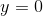andStatement 2: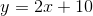and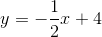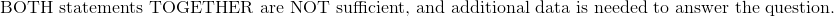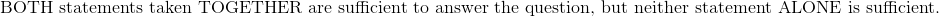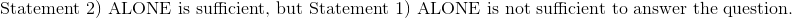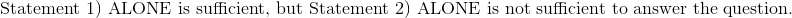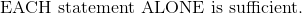Explanation:

Statement 1:andThe lineis a horizontal line on the x-axis.  The lineis a vertical line graphed along the y-axis.  The lines will create perpendicular angles, which are all 90 degrees.

Statement 2:andThese two functions are inform, which allows us to determine the slopes of these functions.  The slopes are 2 and negative half, which are both the negative reciprocal to each other.  The property of negative reciprocal slopes state that these two lines are also perpendicular to each other.

Therefore:### Example Question #4 : Dsq: Understanding Intersecting Lines

Determine the value of the four angles created by the intersecting lines.

Statement 1:  Two angles are acute, and two angles are obtuse.

Statement 2:  Any two non-perpendicular intersecting lines with known equations.Explanation:

Statement 1):  Two angles are acute, and two angles are obtuse.

This statement is not necessarily true.  Two intersecting lines may also be perpendicular to each other, which means that all four angles are 90 degrees.

There is not enough information to justify this statement.

Statement 2:  Any two non-perpendicular intersecting lines with known equations.

This is a tricky statement.

When two functions meet, they must have an intersecting point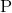.  Both functions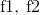can be set equal to each other to determine that intersecting point.

Draw an imaginary line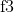where the line is perpendicular to the first function and passes through the second function at some known arbitrary point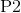. Point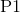will need to be determined.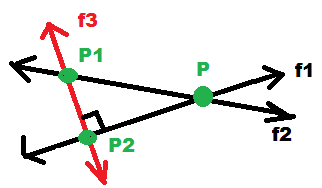The equation of the third function can be determined since imaginary lineintersects equation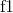at, andis also perpendicular to.  The slope ofcan be determined since it's the negative reciprocal of the slope of.

After the equationhas been determined by using pointand the slope of, the pointcan also be determined by setting the functions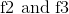equal to each other.

Once the points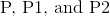have been determined, the distance formula may be used to determine the lengths from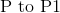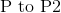, and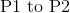.

The Law of Sines can then be used to determine the interior angles of the triangle bounded by.  Knowing one angle at the intersection ofis sufficient to solve for all four angles by supplementary and opposite angle rules.

Therefore:### Example Question #5 : Dsq: Understanding Intersecting Lines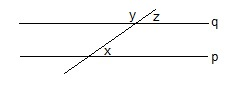What is the value of x?

(1)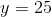(2) Lines p and q are parallel

Statements (1) and (2) TOGETHER are NOT sufficient to answer the question asked, and additional data specific to the problem are needed.

BOTH statements (1) and (2) TOGETHER are sufficient to answer the question asked, but NEITHER statement ALONE is sufficient to answer the question asked.

Statement (1) ALONE is sufficient, but statement (2) alone is not sufficient to answer the question asked.

Statement (2) ALONE is sufficient, but statement (1) alone is not sufficient to answer the question asked.

BOTH statements (1) and (2) TOGETHER are sufficient to answer the question asked, but NEITHER statement ALONE is sufficient to answer the question asked.

Explanation:

With just statement 1, there is no definitive relationship between angle y and angle x.

With just statement 2, there is a difinitive relationship between angle y and angle x, but we don't know the measure of angle y.

If you have the information from both statements 1 and 2, you can determine the measure of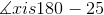, so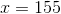.

Fortunately, this is a data sufficiency question, so you don't have to actually do the math, you just have to know that you have all the information to do the math.

### Example Question #6 : Dsq: Understanding Intersecting LinesWhat is the value of x?

(1)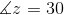(2)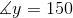Statement (1) ALONE is sufficient, but statement (2) alone is not sufficient to answer the question asked.

BOTH statements (1) and (2) TOGETHER are sufficient to answer the question asked, but NEITHER statement ALONE is sufficient to answer the question asked.

Statements (1) and (2) TOGETHER are NOT sufficient to answer the question asked, and additional data specific to the problem are needed.

Statement (2) ALONE is sufficient, but statement (1) alone is not sufficient to answer the question asked.

Statements (1) and (2) TOGETHER are NOT sufficient to answer the question asked, and additional data specific to the problem are needed.

Explanation:

With just statement 1, we know, so we could determine the measure of angle y, but there is no definitive relationship between z and x nor y and x, because we don't know if lines p and q are parallel.

With just statement 2,we know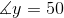, so we could determine the measure of angle z, but there is no definitive relationship between y and x nor z and x, because we don't know if lines p and q are parallel.

Even if we have the information from both statements 1 and 2, we still do not know if lines p and q are parallel, therefore there is no difinitive relationship between angle y and angle x nor angle z and angle x.

### Example Question #7 : Dsq: Understanding Intersecting LinesLines p and q are parallel. What's the value of x?

(1)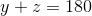(2)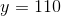BOTH statements (1) and (2) TOGETHER are sufficient to answer the question asked, but NEITHER statement ALONE is sufficient to answer the question asked.

Statements (1) and (2) TOGETHER are NOT sufficient to answer the question asked, and additional data specific to the problem are needed.

Statement (2) ALONE is sufficient, but statement (1) alone is not sufficient to answer the question asked.

Statement (1) ALONE is sufficient, but statement (2) alone is not sufficient to answer the question asked.

With the information from the question that lines p and q are parallel, and the added information from statement 2 that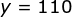, using the rules of supplementary angles and alternate interior angles, we can determine the value of x.